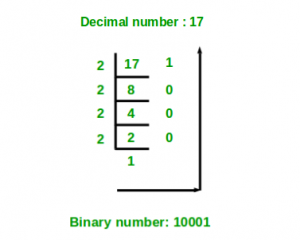Open in App
Not now

# Python program to convert decimal to binary number

• Difficulty Level : Easy
• Last Updated : 30 Dec, 2022

Given a decimal number as input, the task is to write a Python program to convert the given decimal number into an equivalent binary number.
Examples :

```Input : 7
Output :111

Input :10
Output :1010```

Method #1: Recursive solution

```DecimalToBinary(num):
if num >= 1:
DecimalToBinary(num // 2)
print num % 2 ```Below is the implementation of the above recursive solution:

## Python3

 `# Function to convert decimal number``# to binary using recursion``def` `DecimalToBinary(num):``    ` `    ``if` `num >``=` `1``:``        ``DecimalToBinary(num ``/``/` `2``)``    ``print``(num ``%` `2``, end ``=` `'')` `# Driver Code``if` `__name__ ``=``=` `'__main__'``:``    ` `    ``# decimal value``    ``dec_val ``=` `24``    ` `    ``# Calling function``    ``DecimalToBinary(dec_val)`

Output

`011000`

Method #2: Decimal to binary using in-built function

## Python3

 `# Python program to convert decimal to binary``  ` `# Function to convert Decimal number``# to Binary number``def` `decimalToBinary(n):``    ``return` `bin``(n).replace(``"0b"``, "")``  ` `# Driver code``if` `__name__ ``=``=` `'__main__'``:``    ``print``(decimalToBinary(``8``))``    ``print``(decimalToBinary(``18``))``    ``print``(decimalToBinary(``7``))`

Output

```1000
10010
111```

Method #3:Without in-built function

## Python3

 `# Python program to convert decimal to binary``  ` `# Function to convert Decimal number``# to Binary number``def` `decimalToBinary(n):``    ``return` `"{0:b}"``.``format``(``int``(n))``  ` `# Driver code``if` `__name__ ``=``=` `'__main__'``:``    ``print``(decimalToBinary(``8``))``    ``print``(decimalToBinary(``18``))``    ``print``(decimalToBinary(``7``))`

Output

```1000
10010
111```

Quick Ninja Method: One Line Code to Convert Decimal to Binary with user input

## Python3

 `# Quick Ninja One line Code``print``(``bin``(``4785``)[``2``:])`

Output

`1001010110001`

or

## Python3

 `# Use this for user input``#decNum = int(input("Enter any Decimal Number: "))` `decNum ``=` `4785``print``(``bin``(decNum)[``2``:])` `decNum1 ``=` `10``print``(``bin``(decNum1)[``2``:])` `decNum2 ``=` `345``print``(``bin``(decNum2)[``2``:])`

Output

```1001010110001
1010
101011001```

Using the bitwise shift operator >>.

## Python3

 `def` `dec2bin(number: ``int``):``    ``ans ``=` `""``    ``if` `( number ``=``=` `0` `):``        ``return` `0``    ``while` `( number ):``        ``ans ``+``=` `str``(number&``1``)``        ``number ``=` `number >> ``1``    ` `    ``ans ``=` `ans[::``-``1``]` `    ``return` `ans`  `def` `main():``    ``number ``=` `60``    ``print``(f``"The binary of the number {number} is {dec2bin(number)}"``)`  `# driver code``if` `__name__ ``=``=` `"__main__"``:``    ``main()`

Output

`The binary of the number 60 is 111100`

Using built-in format method:

Another approach that is using the built-in format() function. This approach involves converting the decimal number to an integer and then using the format() function with the ‘b’ format specifier to convert it to a binary string. The binary string can then be printed or stored for later use.

Here is an example of how this approach can be used:

## Python

 `def` `decimal_to_binary(decimal_num):``    ``binary_str ``=` `format``(``int``(decimal_num), ``'b'``)``    ``return` `binary_str` `print``(decimal_to_binary(``7``))  ``# prints 111``print``(decimal_to_binary(``10``))  ``# prints 1010` `#This code is contributed by Edula Vinay Kumar Reddy`

Output

```111
1010```

My Personal Notes arrow_drop_up